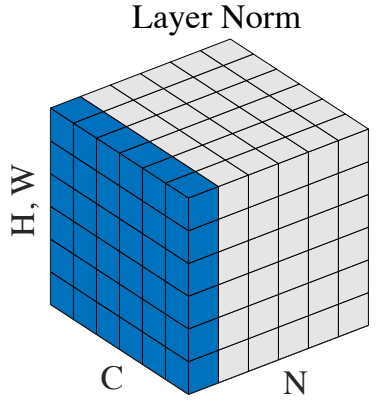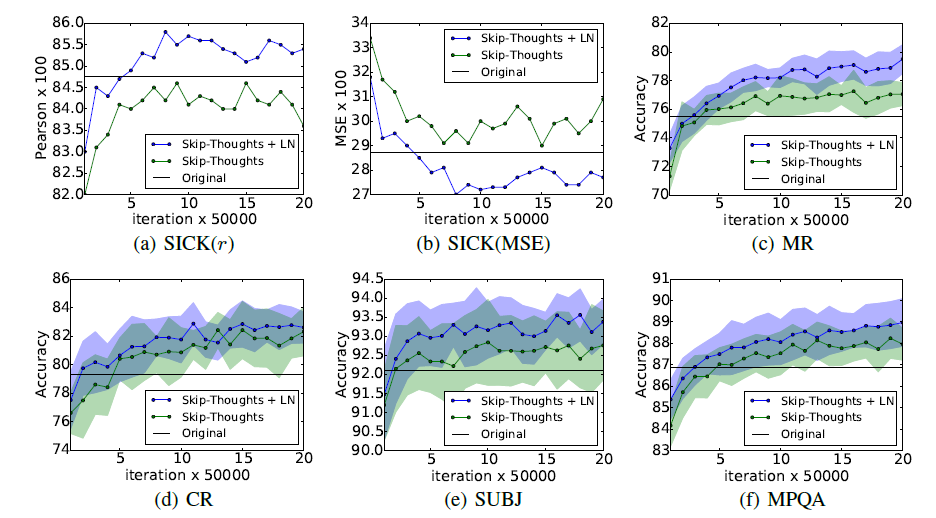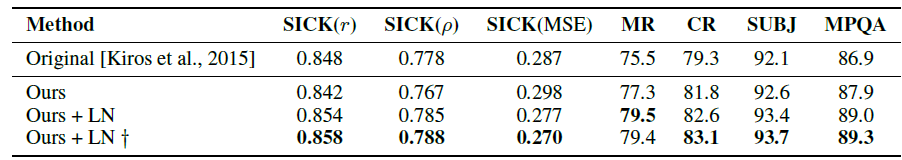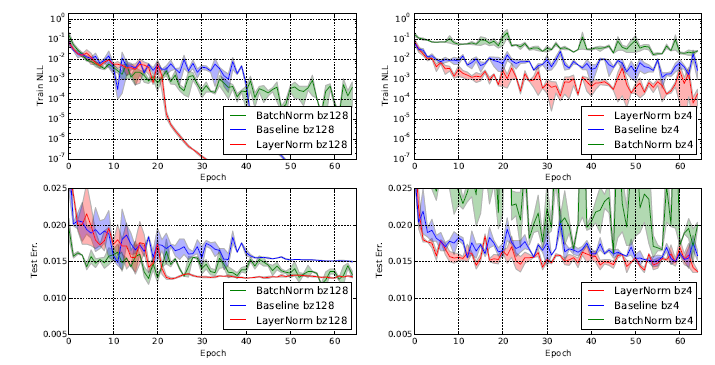# Review: Layer Normalization (LN)

## Stabilizing Training, Reduce Training TimeLayer Normalization (Image from Group Normalization)

Layer Normalization
LN, by University of Toronto, and Google Inc.
2016 arXiv, Over 4000 Citations (Sik-Ho Tsang @ Medium)
Image Classification, Batch Normalization, Layer Normalization

• Batch Normalization (BN) is dependent on the mini-batch size.
• Layer Normalization (LN) is proposed by computing the mean and variance used for normalization from all of the summed inputs to the neurons in a layer on a single training case.
• This is a tech report from Prof. Geoffrey E. Hinton Group.

# Outline

1. Batch Normalization (BN)
2. Layer Normalization (LN)
3. Experimental Results

# 1. Batch Normalization (BN)

## 1.1. Conventional Neural Network Without BN

• A feed-forward neural network is a non-linear mapping from a input pattern x to an output vector y.
• The summed inputs are computed through a linear projection with the weight matrix Wl and the bottom-up inputs hl given as follows:
• where bli is bias.

## 1.2. Conventional Neural Network With BN

• BN was proposed in BN-Inception / Inception-v2 to reduce undesirable “covariate shift”. The method normalizes the summed inputs to each hidden unit over the training cases.
• Specifically, for the i-th summed input in the l-th layer, the batch normalization method rescales the summed inputs according to their variances under the distribution of the data:
• where bar(ali) is normalized summed inputs to the i-th hidden unit in the l-th layer and gi is a gain parameter scaling the normalized activation before the non-linear activation function.
• Note the expectation is under the whole training data distribution.
• μ and σ are estimated using the empirical samples from the current mini-batch.

This puts constraints on the size of a mini-batch and it is hard to apply to recurrent neural networks (RNN).

# 2. Layer Normalization (LN)

## 2.1. LN

• In LN, the “covariate shift” problem can be reduced by fixing the mean and the variance of the summed inputs within each layer.
• The LN statistics is computed over all the hidden units in the same layer as follows:
• where H denotes the number of hidden units in a layer.

Unlike BN, LN does not impose any constraint on the size of a mini-batch and it can be used in the pure online regime with batch size 1.

## 2.2. Layer Normalized RNN

• In a standard RNN, the summed inputs in the recurrent layer are computed from the current input xt and previous vector of hidden states ht-1:

In a standard RNN, there is a tendency for the average magnitude of the summed inputs to the recurrent units to either grow or shrink at every time-step, leading to exploding or vanishing gradients.

• The layer normalized recurrent layer re-centers and re-scales its activations:
• where Whh is the recurrent hidden to hidden weights and Wxh are the bottom up input to hidden weights. ⊙ is the element-wise multiplication between two vectors. b and g are defined as the bias and gain parameters of the same dimension as ht.

LN in RNN results in much more stable hidden-to-hidden dynamics.

# 3. Experimental Results

## 3.1. Skip-Thought ExperimentsPerformance of Skip-Thought with and without LN on downstream tasks as a function of training iterationsSkip-Thought Results

Applying LN results both in speedup over the baseline as well as better final results after 1M iterations.

## 3.2. Permutation Invariant MNISTPermutation invariant MNIST 784–1000–1000–10 model negative log likelihood and test error with layer normalization and batch normalization.
• LN is only applied to the fully-connected hidden layers excluding the last softmax layer.

LN is robust to the batch-sizes and exhibits a faster training convergence comparing to BN that is applied to all layers.

In this paper, CNN is tried but the performance is not as good as fully connected network, and authors said further research is needed to make LN work well in ConvNets.

Yet, later research works already show that LN performs BN when batch size is small, or batches need to be distributed to multiple GPUs.

Layer Norm is used in many NLP models such as Transformer and Transformer-XL.

## Reference

[2016 arXiv] [Layer Norm, LN]
Layer Normalization

## Image Classification

1989–2018 … 2016 [Layer Norm, LN] … 2019: [ResNet-38] [AmoebaNet] [ESPNetv2] [MnasNet] [Single-Path NAS] [DARTS] [ProxylessNAS] [MobileNetV3] [FBNet] [ShakeDrop] [CutMix] [MixConv] [EfficientNet] [ABN] [SKNet] [CB Loss] [AutoAugment, AA] [BagNet] [Stylized-ImageNet] [FixRes] [Ramachandran’s NeurIPS’19] [SE-WRN] [SGELU] [ImageNet-V2]
2020: [Random Erasing (RE)] [SAOL] [AdderNet] [FixEfficientNet]
2021: [Learned Resizer] [Vision Transformer, ViT]

--

--